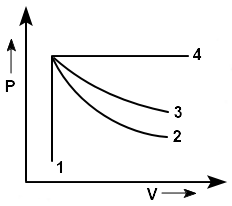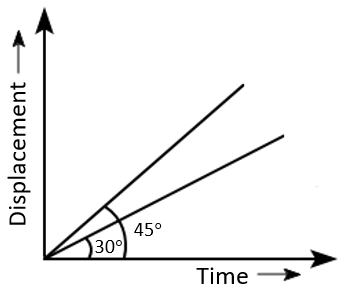Two objects of mass 10 kg and 20 kg respectively are connected to the two ends of a rigid rod of length 10 m with negligible mass. The distance of the center of mass of the system from the 10 kg mass is :
1. 5 m
2. $$\frac{10}{3} \mathrm{~m}$$
3. $$\frac{20}{3} \mathrm{~m}$$
4.  10 m

Subtopic:  Center of Mass |
Please attempt this question first.
Please attempt this question first.
Please attempt this question first.
Launched MCQ Practice Books

Prefer Books for Question Practice? Get NEETprep's Unique MCQ Books with Online Video/Text Solutions via Telegram Bot

Match List - I with List - II:
 List -I (Electromagnetic waves) List - II (Wavelength) (a) AM radio waves (i) $$10^{-10}~m$$ (b) Microwaves (ii) $$10^{2} \mathrm{~m}$$ (c) Infrared radiations (iii) $$10^{-2} \mathrm{~m}$$ (d) X-rays (iv) $$10^{-4} \mathrm{~m}$$
Choose the correct answer from the options given below:
 (a) (b) (c) (d) 1. (ii) (iii) (iv) (i) 2. (iv) (iii) (ii) (i) 3. (iii) (ii) (i) (iv) 4. (iii) (iv) (ii) (i)
Subtopic:  Electromagnetic Spectrum |
Please attempt this question first.
Please attempt this question first.
Please attempt this question first.
Launched MCQ Practice Books

Prefer Books for Question Practice? Get NEETprep's Unique MCQ Books with Online Video/Text Solutions via Telegram Bot

The energy that will be ideally radiated by a 100 kW transmitter in 1 hour is :
1.  1 × 105 J
2.  36 × 107 J
3.  36 × 104 J
4.  36 × 105 J
Subtopic:  Power |
Please attempt this question first.
Please attempt this question first.
Please attempt this question first.
Launched MCQ Practice Books

Prefer Books for Question Practice? Get NEETprep's Unique MCQ Books with Online Video/Text Solutions via Telegram Bot

An ideal gas undergoes four different processes from the same initial state as shown in the figure below. Those processes are adiabatic, isothermal, isobaric and isochoric. The curve which represents the adiabatic process among 1, 2, 3 and 4 is:1. 4
2. 1
3. 2
4. 3
Subtopic:  Types of Processes |
Please attempt this question first.
Please attempt this question first.
Please attempt this question first.
Launched MCQ Practice Books

Prefer Books for Question Practice? Get NEETprep's Unique MCQ Books with Online Video/Text Solutions via Telegram Bot

Plane angle and solid angle have:
1. Both units and dimensions
2. Units but no dimensions
3. Dimensions but no units
4. No units and no dimensions
Subtopic:  Dimensions |
Please attempt this question first.
Please attempt this question first.
Please attempt this question first.
Launched MCQ Practice Books

Prefer Books for Question Practice? Get NEETprep's Unique MCQ Books with Online Video/Text Solutions via Telegram Bot

In half wave rectification, if the input frequency is 60 Hz, then the output frequency would be:
1. 120 Hz
2. zero
3. 30 Hz
4. 60 Hz
Subtopic:  Rectifier |
Please attempt this question first.
Please attempt this question first.
Please attempt this question first.
Launched MCQ Practice Books

Prefer Books for Question Practice? Get NEETprep's Unique MCQ Books with Online Video/Text Solutions via Telegram Bot

When two monochromatic lights of frequency, $$\nu$$ and $$\frac{\nu}{2}$$ are incident on a photoelectric metal, their stopping potential becomes $$\frac{V_{s}}{2}$$ and $$V_s$$, respectively. The threshold frequency for this metal is:
1. $$\frac{3}{2} \nu$$
2. 2$$\nu$$
3. 3$$\nu$$
4. $$\frac{2}{3} \nu$$
Subtopic:  Einstein's Photoelectric Equation |
Please attempt this question first.
Please attempt this question first.
Please attempt this question first.
Launched MCQ Practice Books

Prefer Books for Question Practice? Get NEETprep's Unique MCQ Books with Online Video/Text Solutions via Telegram Bot

The displacement-time graphs of two moving particles make angles of 30° and 45° with the x-axis as shown in the figure. The ratio of their respective velocity is:1. $$1: \sqrt{3}$$
2.$$\sqrt{3}: 1$$
3. 1:1
4.1:2

Subtopic:  Graphs |
Please attempt this question first.
Please attempt this question first.
Please attempt this question first.
Launched MCQ Practice Books

Prefer Books for Question Practice? Get NEETprep's Unique MCQ Books with Online Video/Text Solutions via Telegram Bot

The dimensions [MLT-2A-2] belong to the:
1. electric permittivity
2. magnetic flux
3. self-inductance
4. magnetic permeability
Subtopic:  Dimensions |
Please attempt this question first.
Please attempt this question first.
Please attempt this question first.
Launched MCQ Practice Books

Prefer Books for Question Practice? Get NEETprep's Unique MCQ Books with Online Video/Text Solutions via Telegram Bot

The peak voltage of the ac source is equal to:
1.$$1 / \sqrt{2}$$ times the rms value of the ac source
2. the value of voltage supplied to the circuit
3. the rms value of the ac source
4. $$\sqrt{2}$$ times the rms value of the ac source
Subtopic:  RMS & Average Values |
Please attempt this question first.
Please attempt this question first.
Please attempt this question first.
Launched MCQ Practice Books

Prefer Books for Question Practice? Get NEETprep's Unique MCQ Books with Online Video/Text Solutions via Telegram Bot